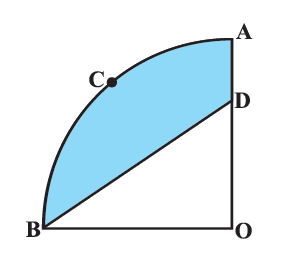# Ex.12.3 Q12 Areas Related to Circles Solution - NCERT Maths Class 10

Go back to  'Ex.12.3'

## Question

In Figure, $${OACB}$$ is a quadrant of a circle with centre $${O}$$ and radius $$3.5\; \rm{cm.}$$ If $$OD = 2 \,\rm{cm,}$$ find the area of the

(i) Quadrant $${OACB,}$$Video Solution
Areas Related To Circles
Ex 12.3 | Question 12

## Text Solution

What is known?

$${OACB}$$ is a quadrant of a circle with centre $${O}$$ and radius $$(r) = \rm{3.5 cm}$$

What is unknown?

(i) Area of the quadrant $${OACB}$$

(ii) Area of the shaded region

Reasoning:

(i) Since quadrant mean \begin{align}\frac{1}{4}\rm{th}\end{align} part.

Therefore  angle  at the centre of a quadrant of a circle\begin{align} = \frac{{360}}{4} = {90^ \circ }\end{align}

Area of the quadrant OACB \begin{align} = \frac{1}{4}\pi {r^2}\end{align}

We can get area of quadrant $${OACB}$$ with radius  $$r = \text{3.5 cm}$$

(ii) Visually from the figure it is clear that

Area of shaded region $$=$$ Area of quadrant $$OACB\; –$$ Area of $$\Delta {BDO}$$

Since $$\angle {BOD} = {90^ \circ }$$

$$\therefore\;$$ For side OB of $$\Delta {BDO, OD}$$  is the

Using formula Area of triangle \begin{align} = \frac{1}{2} \times {\text{base}} \times {\text{height}}\end{align}

We can find Area of $$\Delta {BDO}$$ with base $$= OB = \text{3.5 cm}$$ (radius of quadrant) height $$= OD = \text{2 cm}$$

Steps:

(i)  Since $${OACB}$$ is a quadrant, it will subtend \begin{align} \text{} \,\, \theta = \frac{{{{360}^\circ }}}{4} = {90^\circ }\end{align} angle at $${O}$$

Radius $$(r) = \text{3.5 cm}$$

Area of quadrant $${OACB}$$ \begin{align}= \frac{1}{4}\pi {r^2}\end{align}

\begin{align}&= \frac{1}{4} \times \frac{{22}}{7} \times {\left( {3.5cm} \right)^2}\\&= \frac{1}{4} \times \frac{{22}}{7} \times \frac{7}{2} \times \frac{7}{2}c{m^2}\\&= \frac{{77}}{8}c{m^2}\end{align}

In

\begin{align}\Delta BDO,OB &= r = 3.5cm = \frac{7}{2}cm\\OD &= 2cm\,\angle BOD = {90^{\rm{\circ}}}\end{align}

\begin{align}{\rm{Area of }}\Delta BDO &= \frac{1}{2} \times {\rm{ }}base{\rm{ }} \times height\\&= \frac{1}{2} \times OB \times OD\\& = \frac{1}{2} \times \frac{7}{2}cm \times 2cm\\&= \frac{7}{2}c{m^2}\end{align}

From figure, it is observed that:

Area of shaded region $$=$$ Area of Quadrant $${OACB} \,-$$ Area of $$\Delta {BDO}$$

\begin{align}&= \frac{{77}}{8} - 3.5\,{\text{c}}{{\text{m}}^2}\\&={ \frac{{77}}{8} - \frac{7}{2}{\text{c}}{{\text{m}}^2}}\\&= {\frac{{77 - 28}}{8}{\text{c}}{{\text{m}}^2}}\\&= {\frac{{49}}{8}{\text{c}}{{\text{m}}^2}}\end{align}

Learn from the best math teachers and top your exams

• Live one on one classroom and doubt clearing
• Practice worksheets in and after class for conceptual clarity
• Personalized curriculum to keep up with school